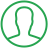# Transpose Of A Matrix In Python

Last Updated : Jul 1, 2023In this tutorial we will show you the solution of transpose of a matrix in python, in python programming, we face various conditions where we have to deal with matrices and their values.

Since matrix is a combination of rows and columns, it plays a very important role in data interpretation.

Since matrix has values in the form of rows and numbers, we’re going to perform this task specifically.

In this tutorial, we’re going to understand the code and the ways of doing transpose of a matrix in python programming.

## Step By Step Guide On Transpose Of A Matrix In Python :-

### Transpose -

• In transpose condition, we just interchange its rows into columns or columns into rows.
• The transpose of the matrix is denoted by using the letter “T” in terms of the mathematics.

We’re going to perform this operation in python language by various methods. Let’s start with the first one.

### Method 1 - By Using Nested List Method

```x = [[5,9],[4,8],[2,9]]
result = [[0, 0, 0], [0, 0, 0]]
for i in range(len(x)):
for j in range(len(x)):
result[j][i] = x[i][j]
for r in Result
print(r)```
1. First, we declared a matrix as its variable ‘x’.
2. Now, we declared a variable named ‘result’ and initialized it with ‘0’ values of the same order of ‘x’ matrix.
3. Then, we applied nested loop and used len() function to return it’s value in the code along with its range in the i(rows) variable.
4. Now for the columns, ‘j’ variable, we used the len() function again and initialised the function value with ‘0’ value.
5. At the end, we called the ‘result’ variable along with the ‘I’ and ‘j’ variables and set this equal to the x[i][j] condition to initialize the values with zero and to get the desired result.
6. Now for the confirmation, we printed the result by using print function after storing the result function as ‘r’ variable.

### Method 2 - Transpose of a Matrix by using Zip () method

Zip function in python, returns single values by making it mapped with the other values within the code. This function is used to map the values having same index which need to show by joined via using a single entity.

```x = [[5,7],[9,4],[6,2]]
res = map(list, zip(*x))
for r in res
print(r)```
1. First we declared a matrix along with values and stored it in the variable ‘x’.
2. Now, we created another variable named ‘res’ and used map(list,zip()) function by passing the matrix variable x.
3. This function will return the matrix’s single values within a single entity in a list form and the star will fulfil the required conditions within the code, it will transpose the matrix.
4. Now, at the end we printed the result ‘res’ within the code for the confirmation and to get the required output.

### Method 3 - Numpy Library Method

Python has its own libraries which has lots of required functions and features. In this method, we will use numpy library functions to perform the task.

```import numpy
x = [[4,5],[6,8],[9,1]]
print(numpy.transpose(x))```
1. Importing numpy library is the very first step here. It’ll make the task easy and reliable to follow up the condition.
2. Now, declared ‘x’ variable has a matrix with its definite values.
3. In this code, we used the transpose function got directly implemented within the print function, so it become short and simple.

## Conclusion :-

In this tutorial, we studies 3 different methods to perform the task of doing transpose of a matrix within the python code.

Every method has their own code and conditions, you can prefer whichever you would like according to the need. I hope this tutorial on transpose of a matrix in python helps you.

##About Amruta

Amruta is an Experienced web developer with 4 years for experience she completed her master's with MCA and passionate about programming Languages for creating technical contents like HTML, CSS, JavaScript, Java, Python, PHP, jQuery.